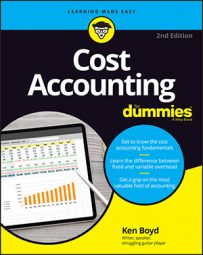##### Cost Accounting For DummiesIn cost accounting practice, a spending variance occurs when the rate or price you pay different from your budget. An efficiency variance is incurred when you use more or less than you plan.

You implement variance analysis to understand differences between planned and actual costs. You hope to learn from the analysis and reduce your costs moving forward.

## Compare the use of the term flexible budget variance in cost accounting

Flexible budget variance is used in the fixed overhead costs section earlier this chapter. However, flexible budget variance has a different meaning for variable overhead. For variable overhead, the flexible budget variance is the sum of two variances.

`Flexible budget variance (also variable overhead variance) = spending variance + efficiency variance`

To keep this straight in your head, flexible budget variance refers to one variance for fixed overhead. Flexible budget variance is the sum of two variances for variable overhead.

## Compute spending variance and efficiency variance in cost accounting

The formula for price variance is:

`Price variance = (actual price - budgeted price) x (actual units sold)`

The price variance formula is similar to the variable overhead spending variance. Instead of actual units sold, this variance uses actual units produced. That’s because you’re applying overhead to production. You use actual and budgeted costs instead of prices. All that being said, here’s the formula:

```Variable overhead spending variance = (\$2.11 - \$1.80) x (18,000)
Variable overhead spending variance = \$5,580```

It’s an unfavorable variance, because you spent more than you planned. Once again, if you get a positive number for a cost variance, it’s unfavorable.

Now consider the variable overhead efficiency variance. If you have an efficiency variance, you used more or less than you planned. You need two calculations for this variance; then you compute the difference between the two amounts.

Here’s the first calculation:

```Actual quantity x budgeted price = 18,000 units x \$1.80
Actual quantity x budgeted price = \$32,400```

The second calculation is budgeted variable overhead applied to actual output.

 Flexible-budget machine hours per output (A) 4 Actual output (units) (B) 5,000 Flexible-budget overhead per machine hour (C) \$1.80 Budgeted variable overhead applied (A x B x C) \$36,000

The variable overhead efficiency variance is the difference between (actual quantity x budgeted price) and (budgeted variable overhead applied to actual output). Here’s the variance:

```Variable overhead efficiency variance = \$32,400 - \$36,000
Variable overhead efficiency variance = (\$3,600).```

Because this is a negative cost variance, it’s favorable.

The variable overhead variance is the sum of the spending variance and the efficiency variance. Here’s your variance calculation:

```Variable overhead variance = \$5,580 + (\$3,600)

Because the positive cost variance is unfavorable, that means your costs for variable overhead were more than planned.

One point bears repeating: You have a variance because you spent more or less than planned (the price or rate you paid had a variance), or you used more or less than planned. You can apply that thought to just about any variance in cost accounting.